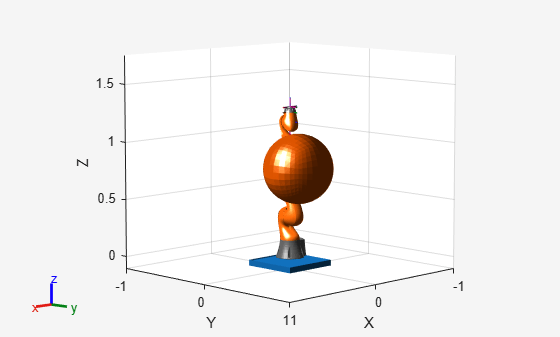# Plan Minimum Jerk Trajectory for Robot Arm

This example shows how to plan a minimum jerk polynomial trajectory for a robotic manipulator. The example shows how to load an included robot model, plan a path for the robot model in an environment with obstacles, generate a minimum jerk trajectory from the path, and visualize the generated trajectories and the robot motion.

### Set Up Robot Model and Environment

This example uses a model of the KUKA LBR iiwa, a 7 degree-of-freedom robot manipulator. Use `loadrobot` to load the robot model into the workspace as a `rigidBodyTree` object. Set the output format for configurations to `"row"`.

`robot = loadrobot("kukaIiwa14","DataFormat","row");`

Generate the environment for the robot. Create collision objects and specify their poses relative to the robot base. Visualize the environment.

```env = {collisionBox(0.5,0.5,0.05) collisionSphere(0.3)}; env{1}.Pose(3,end) = -0.05; env{2}.Pose(1:3,end) = [0.1 0.2 0.8]; show(robot); hold on show(env{1}) show(env{2})```### Plan Path Using manipulatorRRT

Create the RRT planner for the robot model using `manipulatorRRT`. Set the `ValidationDistance` property to increase the number of intermediate states while interpolating the path.

```rrt = manipulatorRRT(robot,env); rrt.ValidationDistance = 0.2; rrt.SkippedSelfCollisions = "parent";```

Specify a start and a goal configuration.

```startConfig = [0.08 -0.65 0.05 0.02 0.04 0.49 0.04]; goalConfig = [2.97 -1.05 0.05 0.02 0.04 0.49 0.04];```

Plan the path. Due to the randomness of the RRT algorithm, set the `rng` seed for repeatability.

```rng(0) path = plan(rrt,startConfig,goalConfig);```

Interpolate the path and retrieve the waypoints.

```interpPath = interpolate(rrt,path); wpts = interpPath';```

### Generate Minimum Jerk Polynomial Trajectory

The planner returns a path as an ordered set of waypoints. To pass these to a robot, you must first determine a trajectory through them. The `minjerkpolytraj` function creates a smooth trajectory with minimum jerk that hits all the specified waypoints.

Provide an initial guess for the times at which the robot arm arrives at the waypoints.

`initialGuess = linspace(0,size(wpts,2)*0.2,size(wpts,2));`

Specify the number of samples to take while estimating the trajectory.

`numSamples = 100;`

Compute the minimum jerk polynomial trajectory.

`[q,qd,qdd,qddd,pp,tpts,tSamples] = minjerkpolytraj(wpts,initialGuess,numSamples);`

### Visualize Trajectories and Waypoints

Plot the trajectories and the waypoints over time.

```minJerkPath = q'; figure plot(tSamples,q) hold all plot(tpts,wpts,"x")```### Visualize Robot Motion

Use the `show` object function to animate the resulting motion. This visualization enables fast updates to ensure a smooth animation.

```figure; ax = show(robot,startConfig); hold all % Ensure the figure pops out of the Live Editor so animations are visible set(gcf,"Visible","on"); for i = 1:length(env) show(env{i},"Parent",ax); end for i = 1:size(minJerkPath,1) show(robot,minJerkPath(i,:),"PreservePlot",false,"FastUpdate",true); drawnow; end hold off```